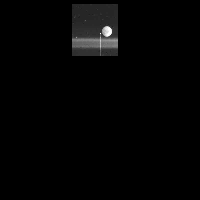# Galileo SSI Image C0416073120

## PDS Label Information:

``````
CCSD3ZF0000100000001NJPL3IF0PDS200000001 = SFDU_LABEL

/* File Format and Length */
RECORD_TYPE = FIXED_LENGTH
RECORD_BYTES = 1000
FILE_RECORDS = 812

/* Pointers to Data Objects */
^TELEMETRY_TABLE = ("C0416073120R.IMG",3)
^IMAGE = ("C0416073120R.IMG",13)
^LINE_PREFIX_TABLE = ("C0416073120R.IMG",13)

/* Description/Catalog Keywords */
DATA_SET_ID = "GO-J/JSA-SSI-2-REDR-V1.0"
SPACECRAFT_NAME = "GALILEO ORBITER"
INSTRUMENT_NAME = "SOLID STATE IMAGING SYSTEM"

/* Time tags and observation descriptors */
SPACECRAFT_CLOCK_CNT_PARTITION = 1
SPACECRAFT_CLOCK_START_COUNT = "04160731.20"
IMAGE_TIME = 1997-10-05T21:18:16.105Z
IMAGE_ID = 10E0102
ORBIT_NUMBER = 10
OBSERVATION_ID = "10ESEURPLM01"
TARGET_NAME = "EUROPA"
NTV_TIME_FROM_CLOSEST_APPROACH = "016T22:07:16Z"
NTV_SAT_TIME_FROM_CLOSEST_APRH = "016T15:57:12Z"
NOTE = "NOT APPLICABLE"

/* Camera and spacecraft state parameters */
FILTER_NAME = "CLEAR"
FILTER_NUMBER = 0
EXPOSURE_DURATION =    62.50
GAIN_MODE_ID = "10K"
FRAME_DURATION =    8.667
LIGHT_FLOOD_STATE_FLAG = "OFF"
EXPOSURE_TYPE = "EXTENDED"
BLEMISH_PROTECTION_FLAG = "OFF"
INVERTED_CLOCK_STATE_FLAG = "NOT INVERTED"
ON_CHIP_MOSAIC_FLAG = "Y"
COMPRESSION_TYPE = "INTEGER COSINE TRANSFORM"
ENTROPY =    1.619
TELEMETRY_FORMAT_ID = "IM8"
OBSTRUCTION_ID = "NOT POSSIBLE"
INSTRUMENT_MODE_ID = "N/A"

/* Viewing Geometry */
/* Note: These viewing geometry parameters are best estimates */
/* at the time this picture label was generated. */
POSITIVE_LONGITUDE_DIRECTION = WEST

/* Spacecraft Geometry */
TARGET_CENTER_DISTANCE = 7.253924e+06
CENTRAL_BODY_DISTANCE = 6.607160e+06
SUB_SPACECRAFT_LATITUDE =   -0.233
SUB_SPACECRAFT_LONGITUDE =  348.078
SUB_SPACECRAFT_LINE =  118.673
SUB_SPACECRAFT_LINE_SAMPLE =  421.923
SUB_SPACECRAFT_AZIMUTH =   94.456

/* Camera and Lighting Geometry                        */
/* Resolution of HORIZONTAL_PIXEL_SCALE,               */
/* VERTICAL_PIXEL_SCALE, and SLANT_DISTANCE            */
/* is calculated from the light source values in       */
/* INTERCEPT_POINT_LATITUDE, INTERCEPT_POINT_LONGITUDE,*/
/* INTERCEPT_POINT_LINE and INTERCEPT_POINT_LINE_SAMPLE keywords  */
/* If the target is a Ring keyword RING_RADIUS         */
/* is substituted for INTERCEPT_POINT_LATITUDE         */
/* If the TARGET_NAME = J RINGS, viewing geometry was */
/* calculated using Jupiter as the target. */
TWIST_ANGLE =   69.056
CONE_ANGLE =   91.888
RIGHT_ASCENSION =  143.273
DECLINATION =   15.208
NORTH_AZIMUTH =   90.424
SMEAR_AZIMUTH = "UNK"
SMEAR_MAGNITUDE = "UNK"
HORIZONTAL_PIXEL_SCALE = 7.364970e+04
VERTICAL_PIXEL_SCALE = 7.363250e+04
SLANT_DISTANCE = 7.252360e+06
SOLAR_DISTANCE = 7.541260e+08
SUB_SOLAR_LATITUDE =    0.673
SUB_SOLAR_LONGITUDE =  166.679
SUB_SOLAR_AZIMUTH =   17.877
INCIDENCE_ANGLE =  178.533
EMISSION_ANGLE =    0.000
PHASE_ANGLE =  178.533
LOCAL_HOUR_ANGLE =  358.601
INTERCEPT_POINT_LATITUDE =   -0.233
INTERCEPT_POINT_LONGITUDE =  348.078
INTERCEPT_POINT_LINE = 118.5
INTERCEPT_POINT_LINE_SAMPLE = 421.9

/* Processing parameters */
MEAN_REFLECTANCE = "N/A"
REFLECTANCE_SCALING_FACTOR = "N/A"
UNEVEN_BIT_WEIGHT_CORR_FLAG = "N/A"
DARK_CURRENT_FILE_NAME = "N/A"
SLOPE_FILE_NAME = "N/A"
BLEMISH_FILE_NAME = "N/A"
SHUTTER_OFFSET_FILE_NAME = "N/A"
DATA_TYPE = LSB_UNSIGNED_INTEGER
SOURCE_PRODUCT_ID = {"S980127A.BSP","S980127A.BSP","N/A","CKMN35AA.PLT","NULL"
}
PROCESSING_HISTORY_TEXT = "VICAR programs run: SSIMERGE,UNMOSAIC,CATLABEL,BADL
ABELS."
PRODUCT_TYPE = "REDR"

/* ICT or Lossless compression */
ENCODING_COMPRESSION_RATIO =   15.666
ENCODING_MIN_COMPRESSION_RATIO =    8.331
ENCODING_MAX_COMPRESSION_RATIO =   40.315
HUFFMAN_TABLE_TYPE = "SKEWED"
ICT_DESPIKE_THRESHOLD = 255
CUT_OUT_WINDOW = {17,289,208,183}
TRUTH_WINDOW = {801,801,96,96}
PRODUCT_VERSION_ID = 0
ICT_QUANTIZATION_STEP_SIZE = 8
ICT_ZIGZAG_PATTERN = "ZIGZAG"
CMPRS_QUANTZ_TBL_ID = "UNIFORM"

/* VICAR IMAGE HEADER Object */
INTERCHANGE_FORMAT = BINARY
BYTES = 2000
RECORDS = 2
^DESCRIPTION = "VICAR2.TXT"
END_OBJECT

/* Table Object (for telemetry table) */
OBJECT = TELEMETRY_TABLE
INTERCHANGE_FORMAT = BINARY
ROWS = 1
COLUMNS = 86
ROW_BYTES = 1800
^STRUCTURE = "RTLMTAB.FMT"
END_OBJECT

INTERCHANGE_FORMAT = BINARY
BYTES = 8000
RECORDS = 8
END_OBJECT

/* Image Object */
OBJECT = IMAGE
LINES = 800
LINE_SAMPLES = 800
SAMPLE_BITS = 8
SAMPLE_TYPE = UNSIGNED_INTEGER
INVALID_CONSTANT = "N/A"
LINE_PREFIX_BYTES = 200
^LINE_PREFIX_STRUCTURE = "RLINEPRX.FMT"
END_OBJECT
END
``````